# Regression analysis

A branch of mathematical statistics that unifies various practical methods for investigating dependence between variables using statistical data (see Regression). The problem of regression in mathematical statistics is characterized by the fact that there is insufficient information about the distributions of the variables under consideration. Suppose, for example, that there are reasons for assuming that a random variablehas a given probability distribution at a fixed valueof another variable, so that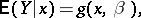whereis a set of unknown parameters determining the function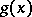, and that it is required to determine the values of these parameters from results of observations. Depending on the nature of the problem and the aims of the analysis, the results of an experiment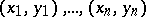are interpreted in different ways in relation to the variable. To ascertain the connection between the variables in the experiment, one often uses a model based on simplified assumptions:is a controllable variable, whose values are given in advance for the design of the experiment, and the observed valuecan be written in the form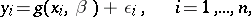where the variables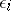characterize the errors, which are independent for various measurements and identically distributed with mean zero and constant variance. In the case of an uncontrollable variable, the results of the observationsrepresent a sample from a certain two-dimensional aggregate. The methods of regression analysis are the same in both cases, although the interpretations of the results differ (in the latter case, the analysis is substantially supplemented by methods from the theory of correlation (in statistics)).

The study of regression for experimental data is carried out using methods based on the principles of mean-square regression. Regression analysis solves the following fundamental problems: 1) the choice of a regression model, which implies assumptions about the dependence of the regression function onand; 2) an estimate of the parametersin the selected model, perhaps by the method of least squares; and 3) testing the statistical hypotheses about the regression.

From the point of view of a single method for estimating unknown parameters, the most natural one is a regression model that is linear in these parameters: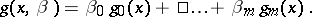The choice of the functionsis sometimes arrived at by arranging the experimental values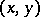on a scattergram or, more often, by theoretical considerations. It is thus assumed that the varianceof the results of the observations is constant (or proportional to a known function of). The standard method of regression estimation is based on the use of a polynomial of some degree,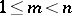: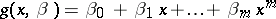or, in the simplest case, of a linear function (linear regression)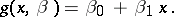There are criteria for testing linearity and for choosing the degree of the approximating polynomial.

According to the principles of mean-square regression, the estimation of the unknown regression coefficients(cf. Regression coefficient) and the variance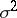(cf. Dispersion) is realized by the method of least squares. Thus, as statistical estimators of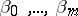one chooses values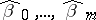which minimize the expressionThe polynomial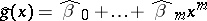thus obtained is called the empirical regression curve, and is a statistical estimator of the unknown proper regression curve. Assuming linearity of regression, the equation of the empirical regression curve has the form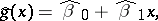where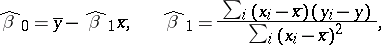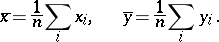The random variablesare called sample regression coefficients (or estimated regression coefficients). An unbiased estimator of the parameteris given by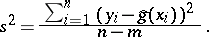If the variance depends on, the method of least squares is applicable with certain modifications.

If one studies the dependence of a random variableon several variables, then it is more convenient to write the general linear regression model in matrix form: An observation vectorwith independent componentshas mean value and covariance matrix given by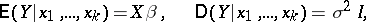(*)

where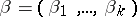is the vector of regression coefficients,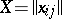,,, is a matrix of known variables related to each other, generally speaking, in an arbitrary fashion, andis the identity matrix of order; moreover,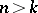and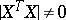. More generally one can assume that there is correlation between the observations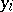: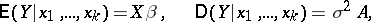for some known matrix. But this scheme can be reduced to the model (*). An unbiased estimator forby the method of least squares is given by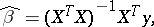and an unbiased estimator foris given byModel (*) is the most general linear model, in that it is applicable to various regression situations and encompasses all forms of polynomial regression ofwith respect to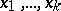(in particular, the above polynomial regression ofwith respect toof ordercan be reduced to the model (*), in whichof the regression variables are functionally connected). In this linear interpretation of regression analysis, the problem of estimatingand the calculation of the covariance matrix of estimatorsreduces to the problem of inverting the matrix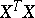.

The above method for constructing an empirical regression assuming a normal distribution of the results of the observations leads to estimators forandthat coincide with the maximum-likelihood estimators. However, the estimators obtained by this method are, in a certain sense, also optimal in the case of deviation from normality, provided only that the sample size is sufficiently large.

In the given matrix form, the general linear regression model (*) admits a simple extension to the case when the observed variablesare random vector variables. This does not give rise to any new statistical problem (see Regression matrix).

The problems of regression analysis are not restricted to the construction of point estimators of the parametersandin the general linear model (*). The problem of the accuracy of a constructed empirical relation is most effectively solved under the assumption that the observation vectoris normally distributed. Ifis normally distributed and since the estimator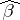is a linear function of, one can conclude that the variable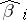is normally distributed with mean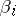and variance, where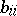is the diagonal entry of the matrix. Apart from this, the estimator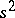foris distributed independently of any component of, and the variablehas a "chi-squared" distribution with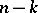degrees of freedom. Hence the statistic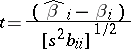has the Student distribution withdegrees of freedom. This fact is used to construct confidence intervals for the parametersand for testing hypotheses about the values taking by them. One can also find confidence intervals for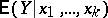for fixed values of all the regression variables, and confidence intervals containing the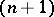-th subsequent value of(called prediction intervals). Finally, starting from a vector of sample regression coefficientsone can construct a confidence ellipsoid for, or for any set of unknown regression coefficients, and also a confidence region for the entire regression curve.

Regression analysis is one of the most widely used methods for processing experimental data when investigating relations in physics, biology, economics, technology, and other fields. Such branches of mathematical statistics as dispersion analysis and the design of experiments are based on models of regression analysis, and these models are widely used in multi-dimensional statistical analysis.